# Conditional probability

(diff) ← Older revision | Latest revision (diff) | Newer revision → (diff)

The conditional probability of an event relative to another event is a characteristic connecting the two events. Ifandare events and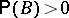, then the conditional probabilityof the eventrelative to (or under the condition, or with respect to)is defined by the equation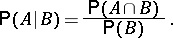The conditional probabilitycan be regarded as the probability that the eventis realized under the condition thathas taken place. For independent eventsandthe conditional probability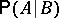coincides with the unconditional probability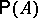.

About the connection between the conditional and unconditional probabilities of events see Bayes formula and Complete probability formula.

The conditional probability of an eventwith respect to a-algebra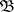is a random variable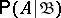, measurable relative to, for which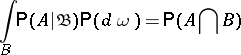for any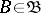. The conditional probability with respect to a-algebra is defined up to equivalence.

If the-algebrais generated by a countable number of disjoint events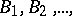having positive probability and the union of which coincides with the whole space, then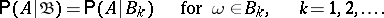The conditional probability of an eventwith respect to the-algebracan be defined as the conditional mathematical expectationof the indicator function of.

Let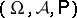be a probability space and letbe a subalgebra of. The conditional probabilityis called regular if there exists a function,,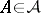, such that

a) for a fixedthe function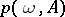is a probability on the-algebra;

b)with probability one.

For a regular conditional probability the conditional mathematical expectation can be expressed by integrals, with the conditional probability taking the role of the measure.

The conditional probability with respect to a random variableis defined as the conditional probability with respect to the-algebra generated by.

How to Cite This Entry:
Conditional probability. Encyclopedia of Mathematics. URL: http://encyclopediaofmath.org/index.php?title=Conditional_probability&oldid=15106
This article was adapted from an original article by V.G. Ushakov (originator), which appeared in Encyclopedia of Mathematics - ISBN 1402006098. See original article